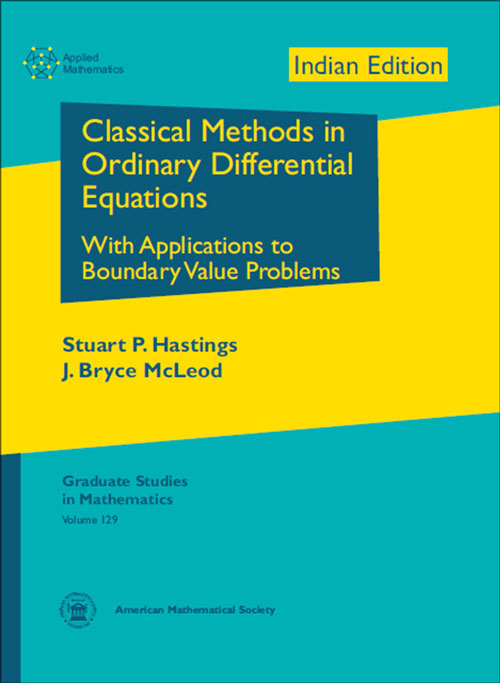Classical Methods in Ordinary Differential Equations: With Applications to Boundary Value Problems
Stuart P. Hastings and J.Bryce McLoed
Price
1600.00
ISBN
9781470409241
Language
English
Pages
392
Format
Paperback
Dimensions
180 x 240 mm
Year of Publishing
2013
Territorial Rights
Restricted
Imprint
Universities Press
Catalogues

This text emphasizes rigorous mathematical techniques for the analysis of boundary value problems for ODEs arising in applications. The emphasis is on proving existence of solutions, but there is also a substantial chapter on uniqueness and multiplicity questions and several chapters which deal with the asymptotic behavior of solutions with respect to either the independent variable or some parameter. These equations may give special solutions of important PDEs, such as steady state or traveling wave solutions. Often two, or even three, approaches to the same problem are described. The advantages and disadvantages of different methods are discussed.

The book gives complete classical proofs, while also emphasizing the importance of modern methods, especially when extensions to infinite dimensional settings are needed. There are some new results as well as new and improved proofs of known theorems. The final chapter presents three unsolved problems which have received much attention over the years.

Both graduate students and more experienced researchers will be interested in the power of classical methods for problems which have also been studied with more abstract techniques. The presentation should be more accessible to mathematically inclined researchers from other areas of science and engineering than most graduate texts in mathematics..

Stuart P. Hastings, University of Pittsburgh, PA, and J. Bryce McLeod, Oxford University, England, and University of Pittsburgh, PA

Preface
Chapter 1. Introduction
1.1. What are classical methods?
1.2. Exercises

Chapter 2. An introduction to shooting methods
2.1. Introduction
2.2. A first order example
2.3. Some second order examples
2.4. Heteroclinic orbits and the FitzHugh-Nagumo equations
2.5. Shooting when there are oscillations: A third order problem
2.6. Boundedness on (-8,8) and two-parameter shooting
2.7. Waz?ewski's principle, Conley index, and an n-dimensional lemma
2.8. Exercises

Chapter 3. Some boundary value problems for the Painlev´e transcendents
3.1. Introduction
3.2. A boundary value problem for Painlev´e
3.3. Painlev´e II—shooting from infinity
3.4. Some interesting consequences
3.5. Exercises

Chapter 4. Periodic solutions of a higher order system
4.1. Introduction, Hopf bifurcation approach
4.2. A global approach via the Brouwer fixed point theorem
4.3. Subsequent developments
4.4. Exercises

Chapter 5. A linear example
5.1. Statement of the problem and a basic lemma
5.2. Uniqueness
5.3. Existence using Schauder's fixed point theorem
5.4. Existence using a continuation method
5.5. Existence using linear algebra and finite dimensional continuation
5.6. A fourth proof
5.7. Exercises

Chapter 6. Homoclinic orbits of the FitzHugh-Nagumo equations
6.1. Introduction
6.2. Existence of two bounded solutions
6.3. Existence of homoclinic orbits using geometric perturbation theory
6.4. Existence of homoclinic orbits by shooting
6.5. Advantages of the two methods
6.6. Exercises

Chapter 7. Singular perturbation problems—rigorous matching
7.1. Introduction to the method of matched asymptotic expansions
7.2. A problem of Kaplun and Lagerstrom
7.3. A geometric approach
7.4. A classical approach
7.5. The case n = 3
7.6. The case n = 2
7.7. A second application of the method
7.8. A brief discussion of blow-up in two dimensions
7.9. Exercises

Chapter 8. Asymptotics beyond all orders

8.1. Introduction
8.2. Proof of nonexistence
8.3. Exercises

Chapter 9. Some solutions of the Falkner-Skan equation
9.1. Introduction
9.2. Periodic solutions
9.3. Further periodic and other oscillatory solutions
9.4. Exercises

Chapter 10. Poiseuille flow: Perturbation and decay
10.1. Introduction
10.2. Solutions for small data
10.3. Some details
10.4. A classical eigenvalue approach
10.5. On the spectrum of D?,R? for large R
10.6. Exercises

Chapter 11. Bending of a tapered rod; variational methods and shooting
11.1. Introduction
11.2. A calculus of variations approach in Hilbert space
11.3. Existence by shooting for p > 2
11.4. Proof using Nehari's method
11.5. More about the case p = 2
11.6. Exercises

Chapter 12. Uniqueness and multiplicity
12.1. Introduction
12.2. Uniqueness for a third order problem
12.3. A problem with exactly two solutions
12.4. A problem with exactly three solutions
12.5. The Gelfand and perturbed Gelfand equations in three dimensions
12.6. Uniqueness of the ground state for ?u - u + u3 = 0
12.7. Exercises

Chapter 13. Shooting with more parameters
13.1. A problem from the theory of compressible flow
13.2. A result of Y.-H. Wan
13.3. Exercise
13.4. Appendix: Proof of Wan's theorem

Chapter 14. Some problems of A. C. Lazer
14.1. Introduction
14.2. First Lazer-Leach problem
14.3. The pde result of Landesman and Lazer
14.4. Second Lazer-Leach problem
14.5. Second Landesman-Lazer problem
14.6. A problem of Littlewood, and the Moser twist technique
14.7. Exercises

Chapter 15. Chaotic motion of a pendulum
15.1. Introduction
15.2. Dynamical systems
15.3. Melnikov's method
15.4. Application to a forced pendulum
15.5. Proof of Theorem 15.3 when d = 0
15.6. Damped pendulum with nonperiodic forcing
15.7. Final remarks
15.8. Exercises

Chapter 16. Layers and spikes in reaction-diffusion equations, I
16.1. Introduction
16.2. A model of shallow water sloshing
16.3. Proofs
16.4. Complicated solutions ("chaos")
16.5. Other approaches
16.6. Exercises

Chapter 17. Uniform expansions for a class of second order problems
17.1. Introduction
17.2. Motivation
17.3. Asymptotic expansion
17.4. Exercise

Chapter 18. Layers and spikes in reaction-diffusion equations, II
18.1. A basic existence result
18.2. Variational approach to layers
18.3. Three different existence proofs for a single layer in asimple case
18.4. Uniqueness and stability of a single layer
18.5. Further stable and unstable solutions, including multiple layers
18.6. Single and multiple spikes
18.7. A different type of result for the layer model
18.8. Exercises

Chapter 19. Three unsolved problems
19.1. Homoclinic orbit for the equation of a suspension bridge
19.2. The nonlinear Schr¨odinger equation
19.3. Uniqueness of radial solutions for an elliptic problem
19.4. Comments on the suspension bridge problem
19.5. Comments on the nonlinear Schr¨odinger equation
19.6. Comments on the elliptic problem and a new existence proof
19.7. Exercises
Bibliography
Index

THE BOOKPOINT (INDIA) PVT. LTD.